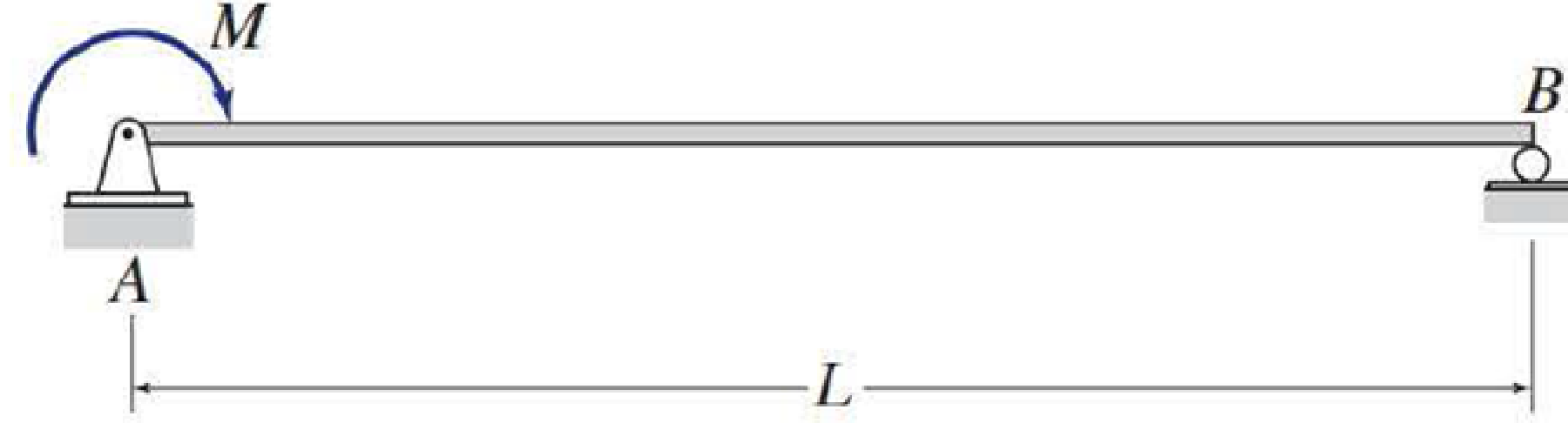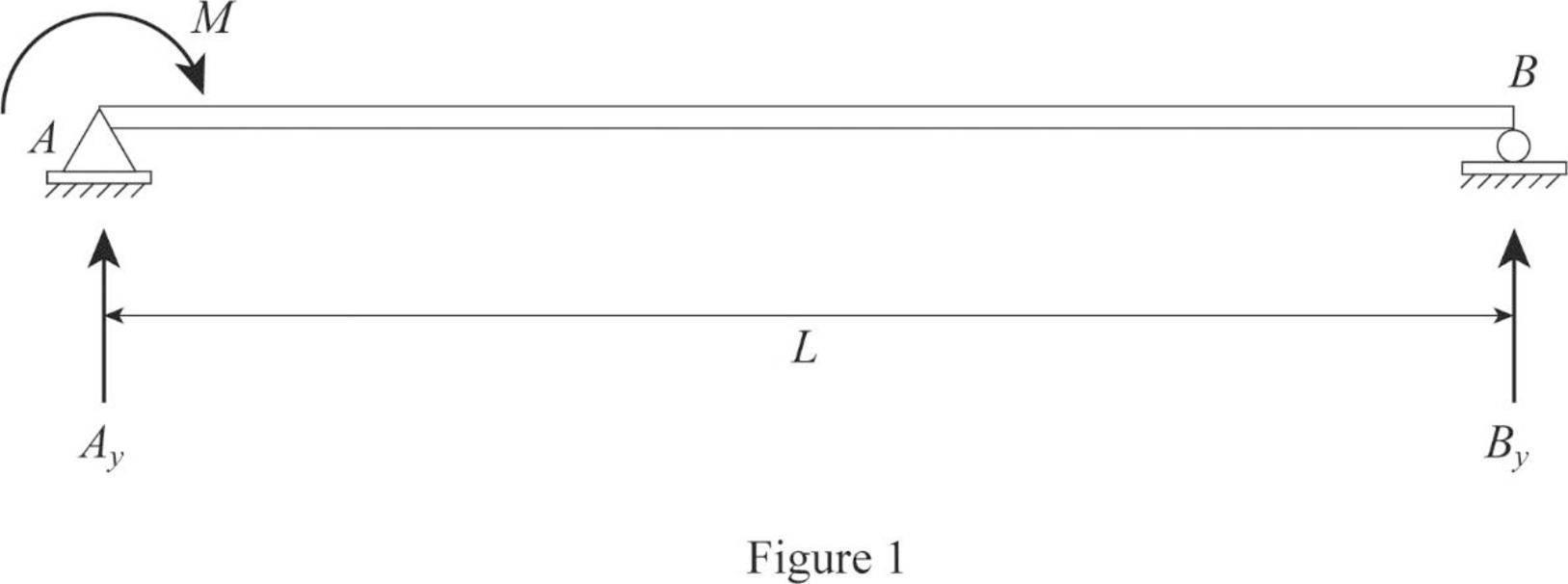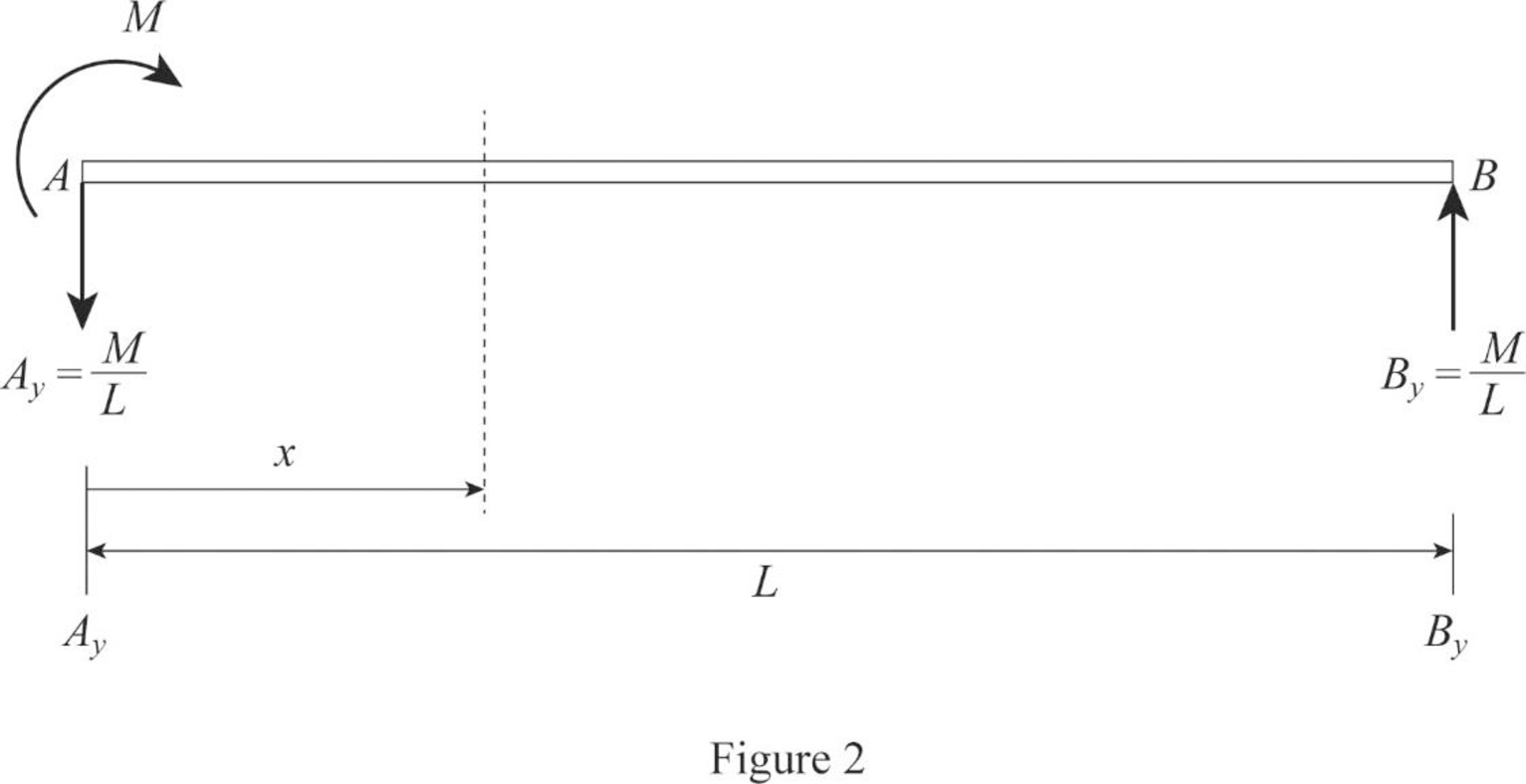# 6.1 through 6.6 Determine the equations for slope and deflection of the beam shown by the direct integration method. EI = constant. FIG. P6.1BuyFind

### Structural Analysis

6th Edition
KASSIMALI + 1 other
Publisher: Cengage,
ISBN: 9781337630931BuyFind

### Structural Analysis

6th Edition
KASSIMALI + 1 other
Publisher: Cengage,
ISBN: 9781337630931

#### Solutions

Chapter 6, Problem 1P
Textbook Problem

## 6.1 through 6.6 Determine the equations for slope and deflection of the beam shown by the direct integration method. EI = constant.FIG. P6.1

Expert Solution
To determine

Find the equations for slope and deflection of the beam using direct integration method.

## Answer to Problem 1P

The equation for slope is M6EIL(3x26Lx+2L2)_.

The equation for deflection is M6EIL[x33Lx2+2L2x]_.

### Explanation of Solution

Calculation:

Consider the flexural rigidity EI of the beam is constant.

Draw the free body diagram of the beam as in Figure (1).Refer Figure (1),

Find the reaction at support B.

MA=0By×LM=0By=ML()

Find the reaction at support A.

MB=0Ay×L+M=0Ay=MLAy=ML()

Draw the section at x distance from support A as in Figure (2).Refer Figure (2),

Write the equation for bending moment at x distance.

Mx=MAy(x)=MML(x)=ML(Lx)

Write the equation for MEI.

d2ydx2=MEI=MEIL(Lx)        (1)

Integrate Equation (1) to find the equation of the slope.

dydx=θ=MEIL(Lx)dx=MEIL(Lxx22)+C1        (2)

Integrate Equation (2) to find the equation of the deflection.

y=[MEIL(Lxx22)+C1]dx=[MEIL(Lx22x36)+C1x+C2]        (3)

Find the integration constants C1andC2:

Apply boundary conditions in Equation (3):

At x=0 and y=0.

0=[MEIL(L×0206)+C1×0+C2]C2=0

At x=L and y=0.

0=[MEIL(L×L22L36)+C1×L+0]0=MEIL(L32L36)+(C1×L)0=ML3EIL(6212)+(C1×L)C1L=ML23EIC1=ML3EI

Find the equation of the slope.

Substitute ML3EI for C1 in Equation (2).

θ=MEIL(Lxx22)ML3EI=M6EIL(6Lx6x22)2L=M6EIL(6Lx3x22L2)=M6EIL(3x26Lx+2L2)

Thus, the equation of the slope is M6EIL(3x26Lx+2L2)_.

Find the equation of the deflection.

Substitute ML3EI for C1 and 0 for C2 in Equation (3).

y=MEIL(Lx22x36)ML3EIx+0=M6EIL[(6Lx226x36)2Lx]=M6EIL[x33Lx2+2L2x]

Thus, the equation of the deflection is M6EIL[x33Lx2+2L2x]_.

### Want to see more full solutions like this?

Subscribe now to access step-by-step solutions to millions of textbook problems written by subject matter experts!

### Want to see more full solutions like this?

Subscribe now to access step-by-step solutions to millions of textbook problems written by subject matter experts!

#### Additional Engineering Textbook Solutions

Find more solutions based on key concepts
Use an elastic analysis and determine the maximum load per inch of weld.

Steel Design (Activate Learning with these NEW titles from Engineering!)

Explain what is meant by pressure and stress and give examples from everyday life.

Engineering Fundamentals: An Introduction to Engineering (MindTap Course List)

Describe how to measure main bearing oil clearance.

Automotive Technology: A Systems Approach (MindTap Course List)

The homogeneous 340-lb sign is suspended from three wires. Find the tension in each wire.

International Edition---engineering Mechanics: Statics, 4th Edition

What is the purpose of a SysSP?

Management Of Information Security

Name uses of cloud storage. Explain criteria for evaluating cloud storage providers.

Enhanced Discovering Computers 2017 (Shelly Cashman Series) (MindTap Course List)

Describe the type(s) of relationship(s) between STORE and REGION.

Database Systems: Design, Implementation, & Management

If your motherboard supports ECC DDR3 memory, can you substitute non-ECC DDR3 memory?

A+ Guide to Hardware (Standalone Book) (MindTap Course List)

Why must the electrode holder be correctly sized?

Welding: Principles and Applications (MindTap Course List)© 2021 bartleby. All Rights Reserved.